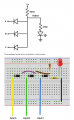# Question about diode AND gate

#### wongojack

Joined Jul 19, 2013
12
Hey AAC - I was working on a project that required a 4 input gate, and wondered if I could simply build it out of a combination of diodes and transistors. I'd never done any work like that before, so I decided to start by building a basic AND gate with diodes. I followed the instructions here: How to Build a Diode AND Gate Circuit (learningaboutelectronics.com)I decided to try and build this as a project with my niece and nephew. It was simple enough, but I was kinda surprised to find out that the AND gate will work as intended even if the cathode end of the diode is NOT connected to a voltage input. This made sense to me as I was building the circuit, but the instructions make a special effort to explain that the voltage has to be the same on the pull up as well as the input, so it kinda surprised me that as long as the inputs are NOT connected to ground that the logic works.

I realize that in an actual device it would only be useful to have the inputs switching between "High" and "Low" and not simply open, but why would the instructions make such a point about matching the voltage when the determining factor seems to be weather or not the cathode end is connected to ground (I used negative)?

I don't know if this is making sense. I'm just curious and thought I would ask this reliable community of experts for their take on this.

#### Papabravo

Joined Feb 24, 2006
20,400
Most of the concepts in digital logic rely on the proposition that there are only two voltage levels. They don't normally deal with unconnected or undefined input levels. If node A for example is not connected to anything then it behaves as "if" it was connected to V+. The reasoning behind this is, that if no current flows through the device, then there can be no voltage across the device. In effect you have converted a 3 input device into a 2-input device, because node A has no effect on the output and it always looks like it is high, TRUE, or 1

The reason they mention this is that there are instances where an unconnected input can cause a real problem in a large and complex system. As you have discovered, a diode AND gate is not one of those places, unless the proper operation of a larger system requires 3 inputs.

Last edited:
•wongojack

#### MrChips

Joined Oct 2, 2009
29,251
The article erred in a number of ways.

The demonstration uses an LED with a current limiting resistor as a test indicator. While a current limiting resistor in series with an LED is always a good idea, in this case the 470Ω resistor is 20 times lower than the 10kΩ pull-up resistor. Hence the 470Ω resistor has little effect.

With 10kΩ pull-up resistor, the max current available to the LED is 5V/10kΩ = 0.5mA which is barely enough to turn on a high efficiency LED. It would make more sense to interchange the two values.

I would have chosen a 1kΩ pullup resistor and a 2.2kΩ LED series resistor.

If you leave the inputs not connected we refer to this as a floating input. In this case, no current flows through the diodes. The full amount of current is available from the pull-up to the output LED. If any diode cathode is connected to ground the current takes the path of lowest resistance and no current flows to the LED. In other words, if the diode is forward biased the LED has no current. Hence what the writer meant was the input diode should not be forward biased for the AND gate to present a logic HI. If the input is connected to Vcc (+5V) then the diode is not forward biased (i.e. not conducting).

In this example it may be simpler to analyze it in terms of current rather than voltage.

•wongojack